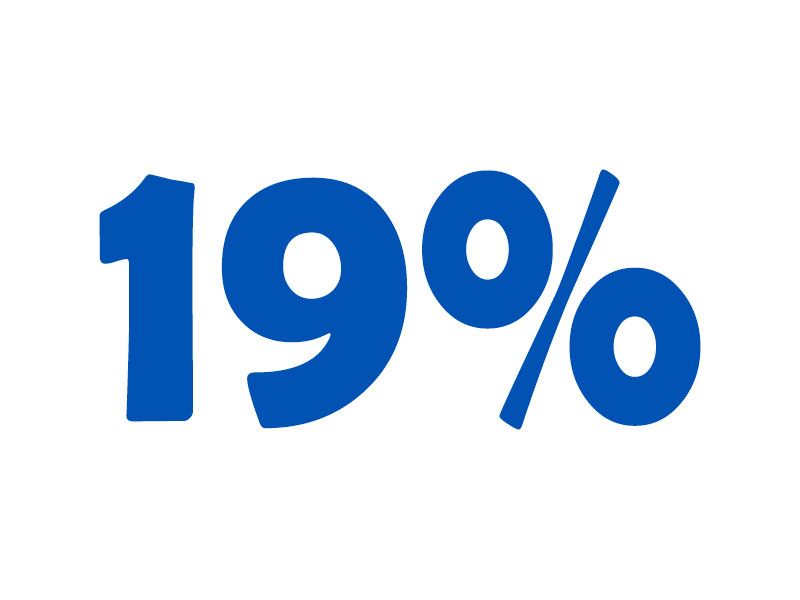Free and Handy Online Calculators2022-07-21T21:26:10+03:00

Welcome! You are on the Calculife.com website. This is a service that allows you to calculate the necessary values ​​online, whether it is volume, length, area, perimeter or something else. You can do all the necessary calculations for free! Use the site search to find the calculator you need, or look below. Handy calculators to simplify your life are here!

•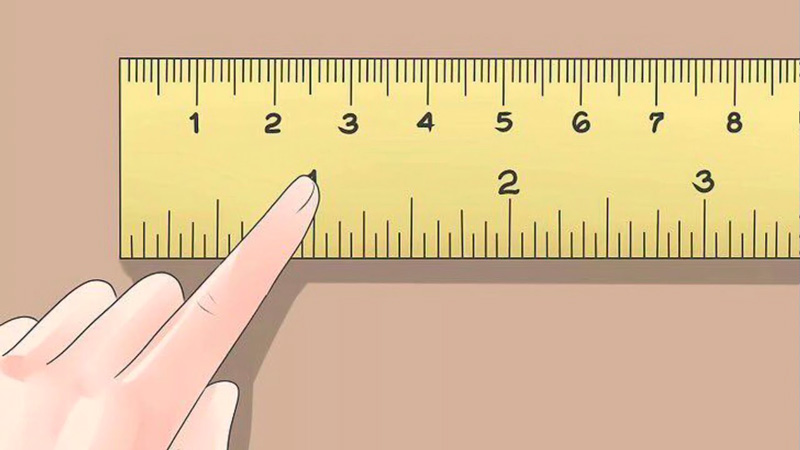## Convert Centimeters to Inches: Handy Online Calculator

•## Convert Pounds to Kilograms: Free Online Calculator

•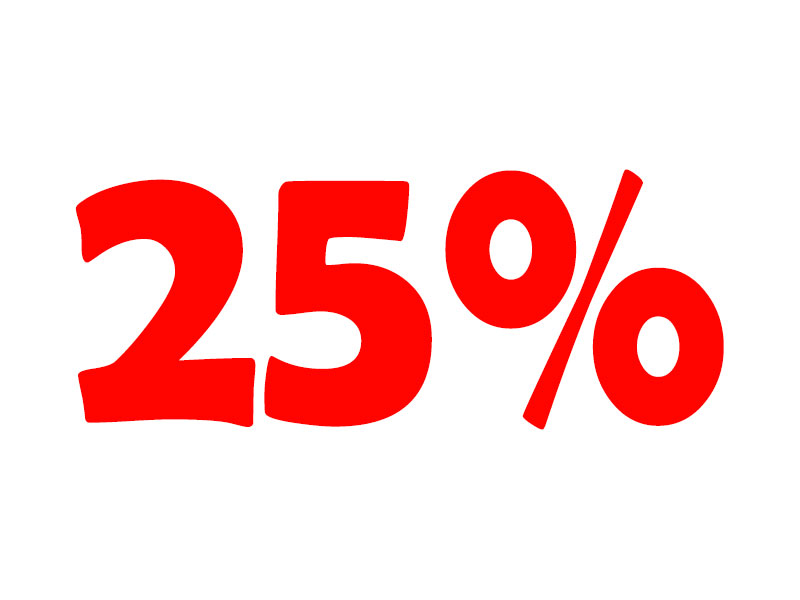## 25% VAT Online Calculator. Add or Subtract 25 Percent Tax

•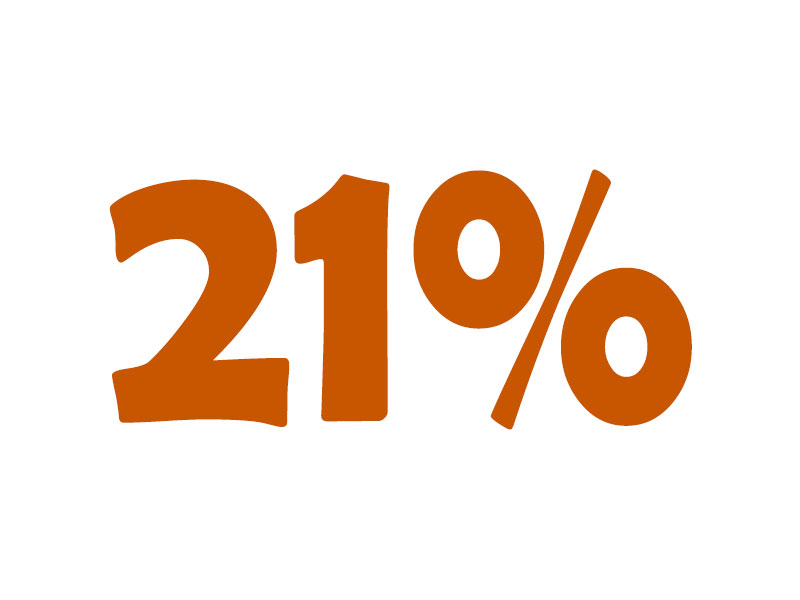## 21% VAT online calculator. Add or subtract 21% tax.

•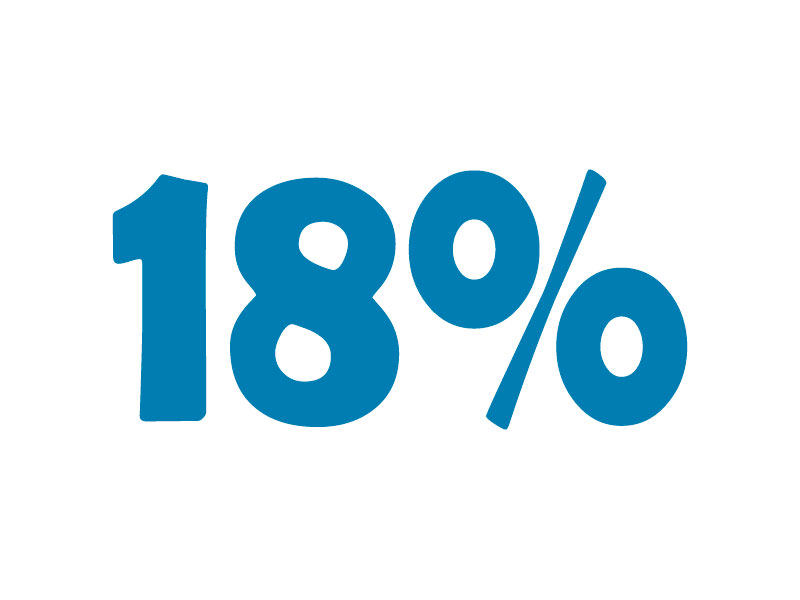## 18% VAT online calculator. Add or subtract 18% tax

•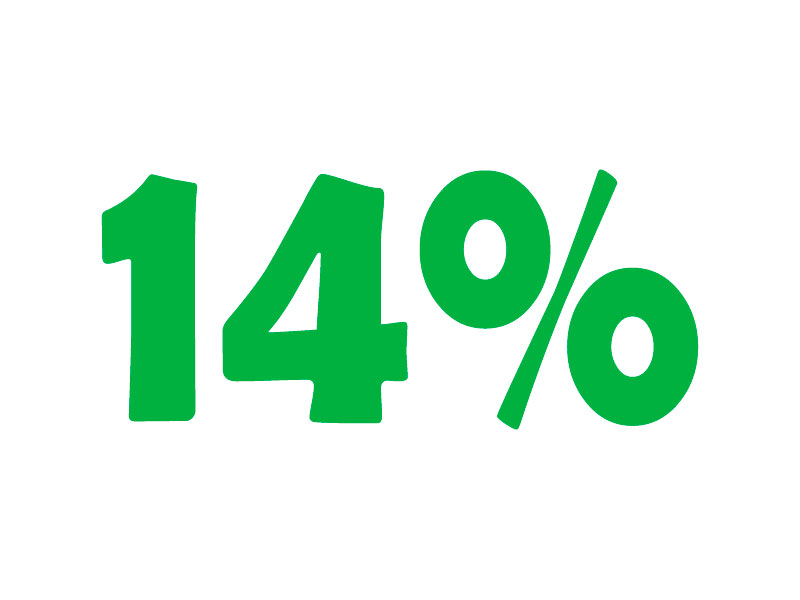## 14% VAT online calculator. Add or subtract 14 percent tax

•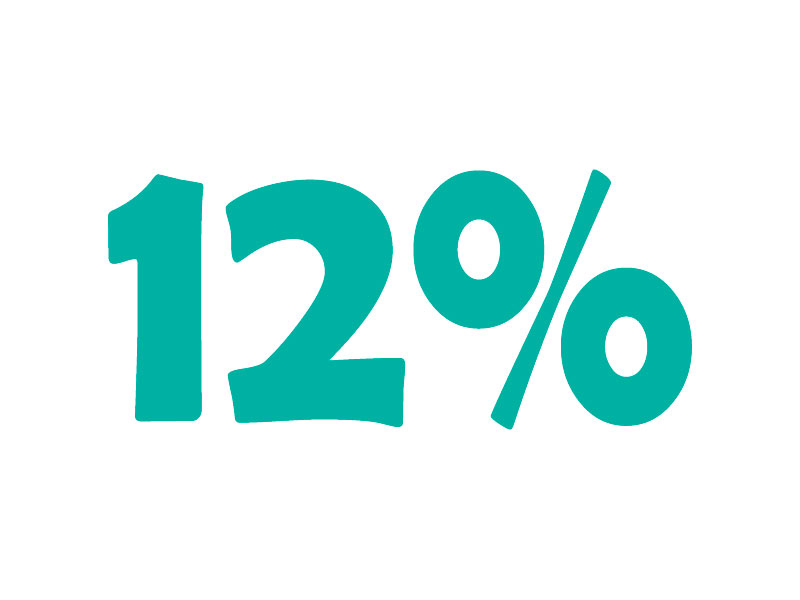## 12% VAT online calculator. Add or subtract 12% tax

•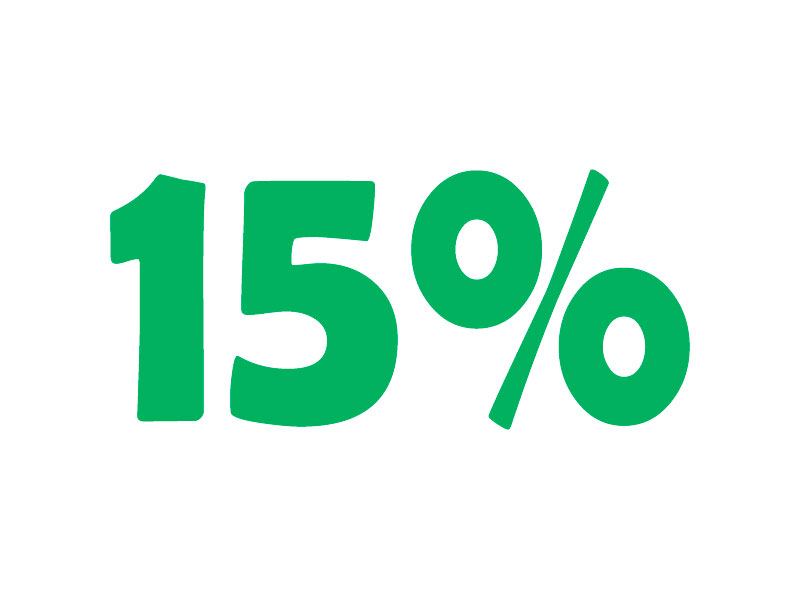## 15% VAT Online Calculator. Add or Subtract 15% Tax

•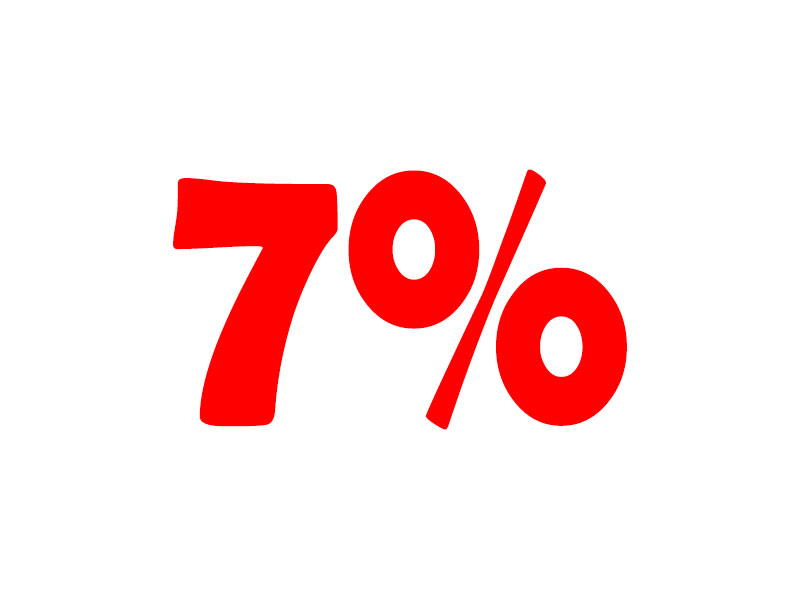## 7% VAT Online Calculator. Add or Subtract 7% Tax

•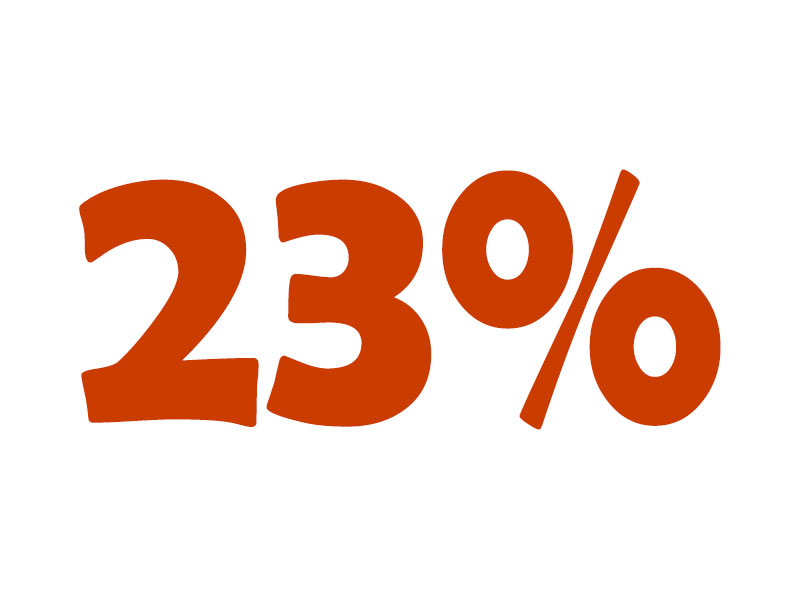## 23% VAT Online Calculator. Add or Subtract 23% Tax

•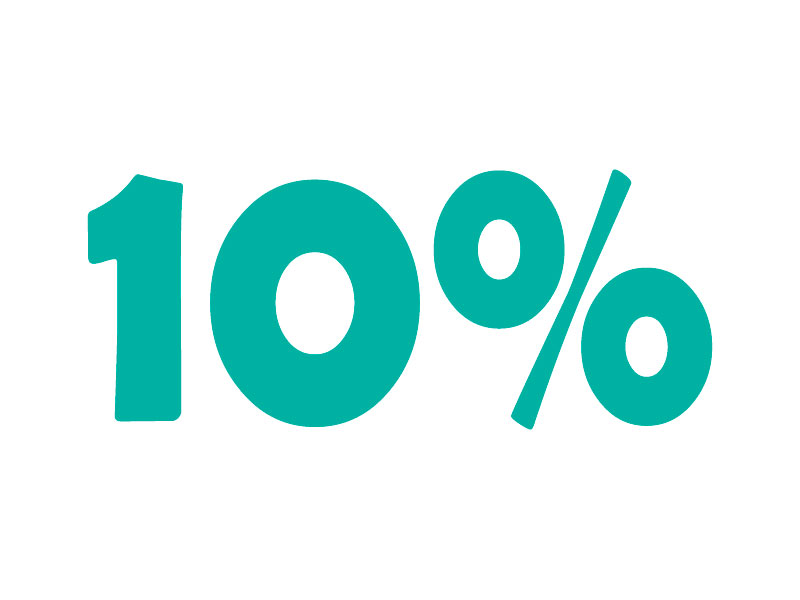## 10% VAT online calculator. Add or subtract 10% tax

•